# DAV Class 3 Maths Chapter 5 Worksheet 8 Solutions

The DAV Class 3 Maths Book Solutions and DAV Class 3 Maths Chapter 5 Worksheet 8 Solutions of Division offer comprehensive answers to textbook questions.

## DAV Class 3 Maths Ch 5 WS 8 Solutions

Find the quotient and the remainder of the following.

Question 1.
76 ÷ 2
Solution: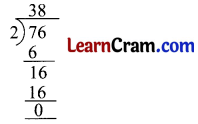Quotient = 38
Remainder = 0

Question 2.
66 ÷ 5
Solution: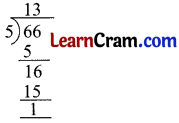Quotient =13
Remainder = 1

Question 3.
92 ÷ 8
Solution:Quotient = 11
Remainder = 4Question 4.
67 ÷ 5
Solution: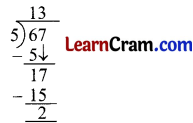Quotient =13
Remainder = 2

Question 5.
75 ÷ 3
Solution:Quotient = 25
Remainder = 0

Question 6.
99 ÷ 7
Solution:Quotient = 14
Remainder = 1Question 7.
89 ÷ 6
Solution:Quotient = 14
Remainder = 5

Question 8.
78 ÷ 2
Solution: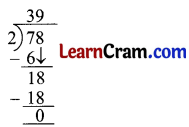Quotient = 39
Remainder = 0

Question 9.
80 ÷ 5
Solution: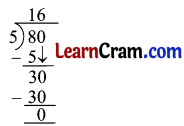Quotient = 16
Remainder = 0### DAV Class 3 Maths Chapter 5 Worksheet 8 Notes

Two-Digit Quotients

Eg., Divide 47 by 4 11Quotient = 11
(Two-digit Quotient)
Remainder = 3
Recall the multiplication table of 4.
7 does not come in table.
The number just less than 7 which comes in table of 4 is 4 itself.